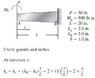• ranaz
In summary, the beam's height at any point is given by the equation h(x) = 2+ (x/L) if x is measured from the point A and the slope of the line through the points (0,ha) and (L,hb) is m.

#### ranaz

This might look a bit stupid but I have just started beams and I can't understand a part in this example it is to do with finding a general equation of the height at any given point on a tapered cantilever beam can someone please explain

#### Attachments

•hx.jpg
10.8 KB · Views: 519
Last edited:
The height of the beam at a distance x from point A is given by the relation h(x) = 2 +(x/L)

You can use this relation to check the given heights of the beam at A and B, which are 2 in and 3 in, respectively.

The relation is already developed and given to you. I suspect the problem is asking you to do something besides developing this relation.

SteamKing said:
The height of the beam at a distance x from point A is given by the relation h(x) = 2 +(x/L)

You can use this relation to check the given heights of the beam at A and B, which are 2 in and 3 in, respectively.

The relation is already developed and given to you. I suspect the problem is asking you to do something besides developing this relation.

I understand that the relationship has been developed, the part I am confused about is that how he has derived this relationship.

If you draw the graph of h against x, it is straight line.

So the relationship is the equation of the straight line through the points (0,ha) and (L,hb).

Plug the values into the standard equation for a line in the form (y-y0) = m(x-x0).

x0 = 0, y0 = ha, and the slope m = (hb-ha)/L.

AlephZero said:
If you draw the graph of h against x, it is straight line.

So the relationship is the equation of the straight line through the points (0,ha) and (L,hb).

Plug the values into the standard equation for a line in the form (y-y0) = m(x-x0).

x0 = 0, y0 = ha, and the slope m = (hb-ha)/L.

OO
Thank you that really helped